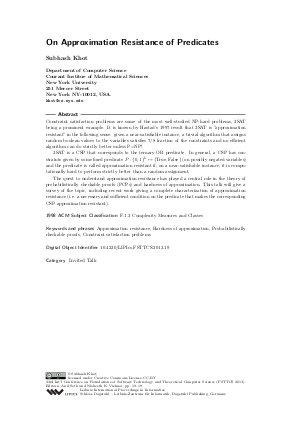Document# On Approximation Resistance of Predicates (Invited Talk)

### Author Subhash Khot## File

LIPIcs.FSTTCS.2013.19.pdf
• Filesize: 213 kB
• 1 pages

## Cite As

Subhash Khot. On Approximation Resistance of Predicates (Invited Talk). In IARCS Annual Conference on Foundations of Software Technology and Theoretical Computer Science (FSTTCS 2013). Leibniz International Proceedings in Informatics (LIPIcs), Volume 24, p. 19, Schloss Dagstuhl - Leibniz-Zentrum für Informatik (2013)
https://doi.org/10.4230/LIPIcs.FSTTCS.2013.19

## Abstract

Constraint satisfaction problems are some of the most well-studied NP-hard problems, 3SAT being a prominent example. It is known by Hastad's 1997 result that 3SAT is "approximation resistant" in the following sense: given a near-satisfiable instance, a trivial algorithm that assigns random boolean values to the variables satisfies 7/8 fraction of the constraints and no efficient algorithm can do strictly better unless P=NP! 3SAT is a CSP that corresponds to the ternary OR predicate. In general, a CSP has constraints given by some fixed predicate P:{0,1}^k -> {True, False} (on possibly negated variables) and the predicate is called approximation resistant if, on a near-satisfiable instance, it is computationally hard to perform strictly better than a random assignment. The quest to understand approximation resistance has played a central role in the theory of probabilistically checkable proofs (PCPs) and hardness of approximation. This talk will give a survey of the topic, including recent work giving a complete characterization of approximation resistance (i.e. a necessary and sufficient condition on the predicate that makes the corresponding CSP approximation resistant).
##### Keywords
• Approximation resistance
• Hardness of approximation
• Probabilistically checkable proofs
• Constraint satisfaction problem

## Metrics

• Access Statistics
• Total Accesses (updated on a weekly basis)
0